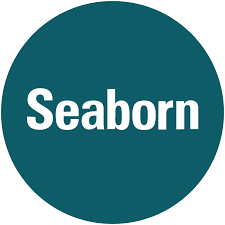# 2.9 - Summary of Seaborn Visualizations

Last updated: March 14th, 2019# Intro to Seaborn¶

Far from being a visualization package created completely from scratch, the goal of seaborn has always been to offer simpler access to the creation of graphics based on matplotlib data. In fact, as indicated in its documentation, seaborn "should be seen as a complement to matplotlib and not a replacement of it."

seaborn depends on matplotlib for the own creation of graphics and only implements a layer of superior abstraction offers:

• Mapping of visual elements from full DataFrame used as input.
• Automation of certain statistical graphics.
• Topics and styles more visually attractive than matplotlib (at least in previous versions).
• Ease of access to color palettes.
• Greater ease for the creation of "multigraphic" graphics.## Hands on!¶

In [ ]:
import numpy as np
import pandas as pd
import matplotlib.pyplot as plt
import seaborn as sns

%matplotlib inline

In [ ]:
iris = sns.load_dataset('iris')

In [ ]:
plt.scatter(iris['sepal_length'], iris['sepal_width'])### Some graphics with seaborn¶

Since seaborn is a complement to matplotlib, the types of graphics it offers are precisely those that matplotlib lacks (or complicates its use). However, since matplotlib continues to evolve, some of the graphics are already part of the library and, over time, it is expected that they will continue to be incorporated.

### One-variable distribution¶

As we have seen, one of the deficiencies that most impact when working with matplotlib is the possibility of showing the distribution of the variables (although we have histograms and, now, of the violin graphics).

Seaborn offers multiple functions that solve this lack for both univariate distributions:

In [ ]:
sns.distplot(iris['sepal_length'],
hist=True,
kde=True,
rug=True)

In [ ]:
sns.kdeplot(iris['sepal_length'])

In [ ]:
sns.rugplot(iris['sepal_length'])### Two-variable distribution¶

Also for bivariate distributions:

In [ ]:
sns.jointplot(x='sepal_length', y='sepal_width', data=iris,
kind='scatter')

In [ ]:
sns.jointplot(x='sepal_length', y='sepal_width', data=iris,
kind='hex')

In [ ]:
sns.jointplot(x='sepal_length', y='sepal_width', data=iris,
kind='kde')### Pairplots, relating two or more variables¶

These plots are used to study the relationship between all the variables with a single visualization.

In [ ]:
sns.pairplot(iris)

In [ ]:
sns.pairplot(iris, vars=['sepal_width', 'petal_length'])

In [ ]:
sns.pairplot(iris, diag_kind='kde')### Regressions¶

Another of the weak points of matplotlib is the inclusion of "statistics" that help to understand the content of a visualization and its underlying dataset directly (since they can always be calculated and included as an additional layer to any graphic).

Seaborn tries to include this functionality to "approximate" that stats.

In [ ]:
sns.lmplot(data=iris, x='sepal_length', y='sepal_width')

In [ ]:
sns.lmplot(data=iris, x='sepal_length', y='sepal_length',
order=3)

In [ ]:
sns.lmplot(data=iris, x='sepal_length', y='sepal_width',
lowess=True)

In [ ]:
sns.lmplot(data=iris, x='sepal_length', y='sepal_width',
hue='species',
markers=["+", "o", "^"])### Charts based on categorical variable¶

We have seen that matplotlib treats ALL the variables included in the charts as numerical sets. If you want to make visualizations based on categorical variables, you need to work with them as numeric and "trick" the content of the axes to give the "feeling" of working with numerical variables.

Seaborn allows the direct use of categorical variables and their correct treatments.

In [ ]:
sns.stripplot(data=iris, x='species', y='sepal_length')

In [ ]:
sns.stripplot(data=iris, x='sepal_length', y='species',
jitter=False)

In [ ]:
sns.stripplot(data=iris, x='sepal_length', y='sepal_width',
hue='species')

In [ ]:
sns.swarmplot(data=iris, x='species', y='sepal_length')

In [ ]:
sns.boxplot(data=iris, x='species', y='sepal_length')

In [ ]:
sns.violinplot(data=iris, x='species', y='sepal_length')### Multigraphic with seaborn¶

Although we have seen that matplotlib allows the creation of multigraphic graphics, we have also talked about the limitations of its generation since they are based solely on positioning and it is the responsibility of the user to adjust scales, the drawing of each graphic in its corresponding position, etc.

In [ ]:
grid = sns.FacetGrid(iris, col='species', hue='species')

grid.map(plt.scatter,'sepal_length', 'sepal_width')


Also, a legend can be added to the grid plots:

In [ ]:
grid = sns.FacetGrid(iris, col='species', hue='species')

grid.map(plt.scatter,'sepal_length', 'sepal_width')

grid.add_legend()

In [ ]:
grid = sns.FacetGrid(iris, row='sepal_width')

grid.map(plt.scatter, 'sepal_length', 'sepal_width')

In [ ]:
diamonds = pd.read_table('data/diamonds.txt', sep=',')

grid = sns.FacetGrid(diamonds, col='cut', row='color')

grid.map(plt.hist, 'price')### Styles in seaborn¶

At the time of the creation of seaborn, the style management of matplotlib was very limited and "forced" its definition based on matplotlibrc files (costly, uncomfortable, inflexible and a priori).

Currently matplotlib has improved a lot in this aspect and the "advantages" offered by seaborn are not so clear.

In [ ]:
sns.axes_style()

In [ ]:
sns.lmplot(data=iris, x='sepal_length', y='sepal_width')

In [ ]:
sns.set_style('white')

sns.lmplot(data=iris, x='sepal_length', y='sepal_width')

In [ ]:
sns.set_style('darkgrid', {
"grid.color": "#cccccc",
"grid.linestyle": "--",
"figure.facecolor": "#eeeeee"})

sns.lmplot(data=iris, x='sepal_length', y='sepal_width')


Top and right borders can be removed using the dispine() function.

In [ ]:
sns.set_style("white")

sns.distplot(iris['sepal_length'])

sns.despine()### Adding title and labels¶

You can also modify title and axis labels in Seaborn.

In [ ]:
sns.distplot(iris['sepal_length'])

In [ ]:
plot = sns.distplot(iris['sepal_length'])

plot.set(title='Sepal length',
xlabel='Value',
ylabel='Frequency')